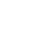# 机器学习100天教程 — 第1天 数据预处理

《机器学习100天》学习计划由 Avik-Jain/100-Days-Of-ML-Code 推出，现在一共有54天的教程，已经积攒了近30k的Star.

## 第0步：安装依赖 #

Windows环境下打开Cmd(开始—运行—CMD)，苹果系统环境下请打开Terminal(command+空格输入Terminal)，输入命令安装依赖：

```pip install numpy
pip install pandas
pip install scikit-learn```

## 第1步：导入库 #

```import numpy as np
import pandas as pd```

## 第2步：导入数据集 #

```dataset = pd.read_csv('Data.csv')//读取csv文件
X = dataset.iloc[ : , :-1].values//.iloc[行，列]
Y = dataset.iloc[ : , 3].values  // : 全部行 or 列；[a]第a行 or 列
// [a,b,c]第 a,b,c 行 or 列
print("Step 2: Importing dataset")
print("X")
print(X)
print("Y")
print(Y)```

## 第3步：处理丢失数据 #

```from sklearn.impute import SimpleImputer
imputer = SimpleImputer(missing_values=np.nan,strategy='mean')
imputer = imputer.fit(X[:,1:3])
X[:,1:3] = imputer.fit_transform(X[:,1:3])
print("---------------------")
print("Step 3: Handling the missing data")
print("step2")
print("X")
print(X)```

## 第4步：解析分类数据 #

```from sklearn.preprocessing import LabelEncoder, OneHotEncoder
from sklearn.compose import ColumnTransformer
labelencoder_X = LabelEncoder()
X[ : , 0] = labelencoder_X.fit_transform(X[ : , 0])

# 创建虚拟变量
onehotencoder = ColumnTransformer([('encoder', OneHotEncoder(), )], remainder='passthrough')
X = onehotencoder.fit_transform(X)
labelencoder_Y = LabelEncoder()
Y =  labelencoder_Y.fit_transform(Y)
print("---------------------")
print("Step 4: Encoding categorical data")
print("X")
print(X)
print("Y")
print(Y)```

## 第5步：拆分数据集为训练集合和测试集合 #

```from sklearn.cross_validation import train_test_split
X_train, X_test, Y_train, Y_test = train_test_split( X , Y , test_size = 0.2, random_state = 0)```

## 第6步：特征缩放 #

```from sklearn.preprocessing import StandardScaler
sc_X = StandardScaler()
X_train = sc_X.fit_transform(X_train)
X_test = sc_X.transform(X_test)```

¥1¥5¥10¥20¥50¥100¥200 自定义

​Python实用宝典 ( pythondict.com )

## 《机器学习100天教程 — 第1天 数据预处理》上的6个想法

1.小新说道：

最新版
from sklearn.impute import SimpleImputer

imputer=SimpleImputer(missing_values=np.nan,strategy=’mean’)
imputer=imputer.fit(X[:,1:3])
X[:,1:3]=imputer.fit_transform(X[:,1:3])

2.小新说道：

最新版报错：

onehotencoder = OneHotEncoder(categorical_features = )

1.Python实用宝典说道：

已更新

3.小新说道：

建议更新，或者在项目前面标注版本，有些都已经变了 ，例如：训练集 from sklearn.model_selection import train_test_split

1.Python实用宝典说道：

感谢提醒，应该是用了旧的版本，我更新一下

2.Python实用宝典说道：

from sklearn.model_selection import train_test_split
应该没问题